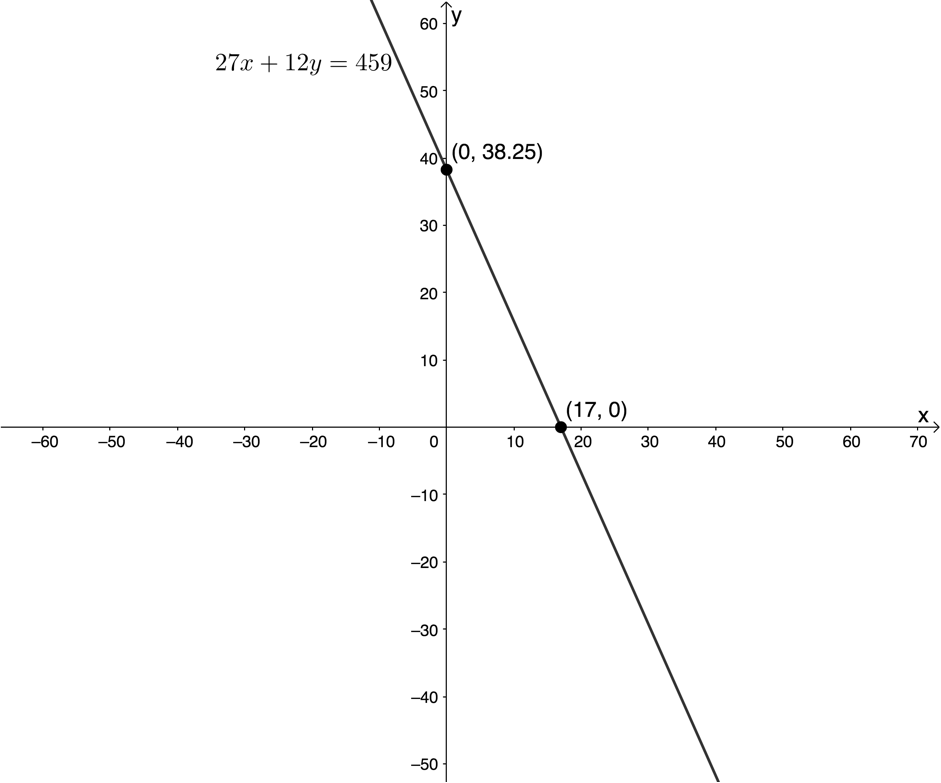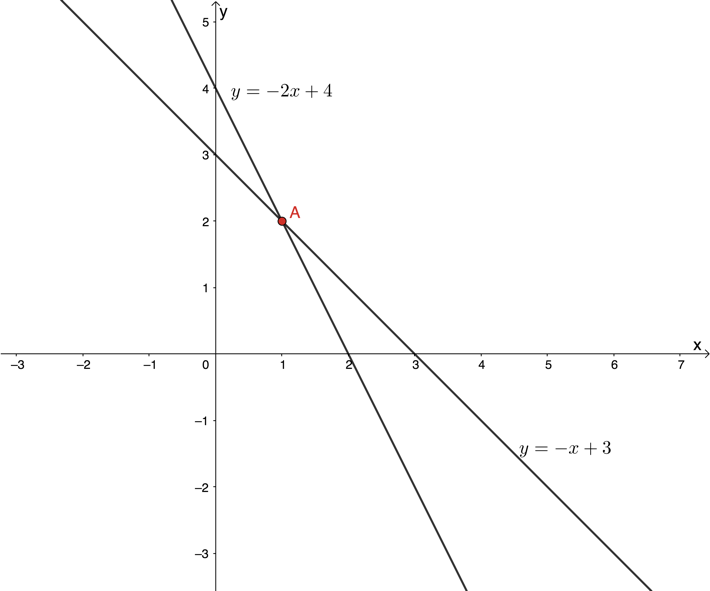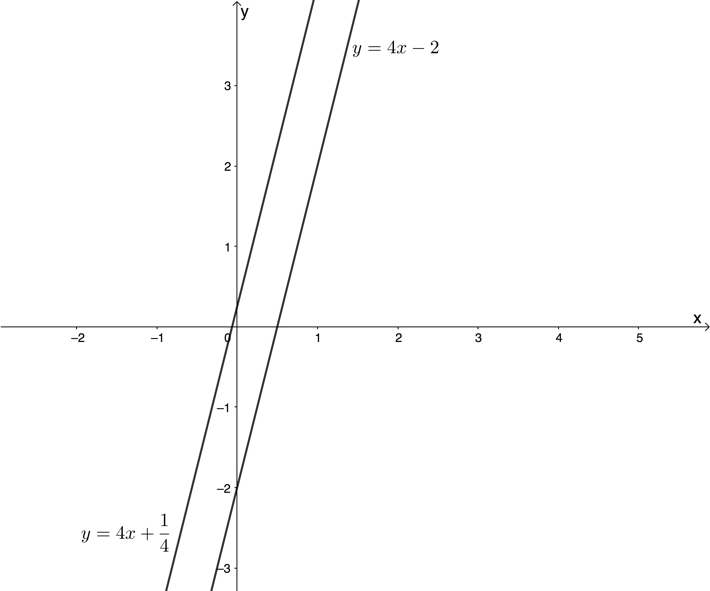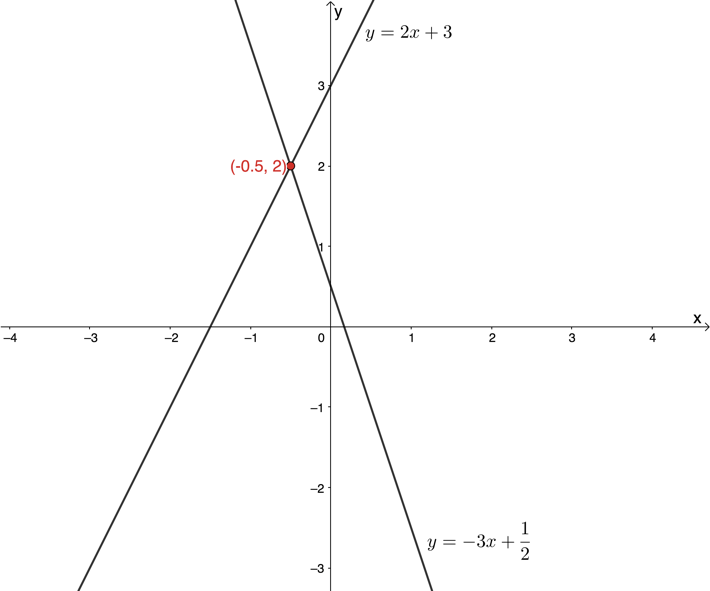Functions and algebra: Solve algebraic equations and inequalities

# Unit 4: Solve simultaneous equations

Dylan Busa### Unit outcomes

By the end of this unit you will be able to:

• Solve systems of simultaneous equations where both equations are linear equations algebraically by means of:
• Elimination
• Substitution.
• Solve systems of simultaneous equations where both equations are linear equations graphically by finding the point of intersection of the functions.

## What you should know

Before you start this unit, make sure you can:

## Introduction

In Unit 1 of this subject outcome, we learnt how to solve linear equations and quadratic equations. We saw that the highest power on the unknown in linear equations is 1 and this corresponds to one solution. The highest power on the unknown in quadratic equations is 2 and this corresponds to two solutions.

In both these cases however, there is still only one unknown and we only need one equation to solve for that unknown. But what if there are two unknowns like in the following case?

A hardware store sells drill bit sets. The BIG set contains $\scriptsize 27$ bits while the SMALL set contains $\scriptsize 12$ bits. In total, a college bought $\scriptsize 459$ bits for its workshops. How many of each set did the college buy?

In this case, we have two unknowns; the number of BIG sets bought, and the number of SMALL sets bought. If we let the number of BIG sets be $\scriptsize x$ and the number of SMALL sets be $\scriptsize y$, we can set up an equation as follows.

$\scriptsize 27x+12y=459$

You probably recognise this as a linear function or a straight-line graph. We can rewrite the equation as $\scriptsize y=\displaystyle \frac{{27}}{{12}}x+\displaystyle \frac{{459}}{{12}}$ ($\scriptsize y=mx+c$ or $\scriptsize y=ax+q$) where the gradient is $\scriptsize \displaystyle \frac{{27}}{{12}}$ and the y-intercept is $\scriptsize \displaystyle \frac{{459}}{{12}}$. If we draw a sketch of the function, we get the graph in Figure 1.Figure 1: Graph of $\scriptsize 27x+12y=459$

We can see from the graph that there are infinitely many possible solutions. All the points that lie on the graph are possible solutions for $\scriptsize x$ and $\scriptsize y$. Which one do we choose? Clearly, we need more information.

Now, what if we know that each BIG set costs $\scriptsize \text{R}68$ and each SMALL set costs $\scriptsize \text{R}42$ and that the college paid a total of $\scriptsize \text{R}1\ 262$ for all the sets? We could set up another equation.

$\scriptsize 68x+42y=1262$

This is also a linear function. What if we drew the graph of this function on the same set of axes as before (see Figure 2)?Figure 2: Graphs of $\scriptsize 27x+12y=459$ and $\scriptsize 68x+42y=1262$

Now we have a single point that lies on both graphs or a single set of values for $\scriptsize x$ and $\scriptsize y$ that satisfies both equations: $\scriptsize x=13$ and $\scriptsize y=9$. Therefore, $\scriptsize 13$ BIG sets and $\scriptsize 9$ SMALL sets were bought.

We can see that in order to solve for two unknowns, we need two equations. If we want to solve for three unknowns, we need three equations. If we want to solve for $\scriptsize n$ unknowns, we need $\scriptsize n$ equations.

We call sets of equations like this simultaneous equations. We will only solve two simultaneous equations at a time, and these will always be linear equations. To do so we have three options: substitution, elimination or graphically (as above).

## Solve simultaneous equations using substitution

The process of solving simultaneous equations by substitution is quite simple.

1. Take one of the equations (usually the simplest) and rearrange it to express one of the variables in terms of the other.
2. Substitute this expression into the other equation. Now we only have one equation with one variable.
3. Solve the equation for this variable.
4. Substitute the solution for this variable back into the originally rearranged equation to solve for the other variable.### Example 4.1

Solve for $\scriptsize x$ and $\scriptsize y$:

$\scriptsize x+y=1$ and $\scriptsize 3=y+2x$

Solution:

It is a very good idea to start by numbering each equation. This makes them easier to refer to in your working.

$\scriptsize x+y=1$ (1)

$\scriptsize 3=y+2x$ (2)

Step 1: Take one of the equations (usually the simplest) and rearrange it to express one of the variables in terms of the other.

From (1):
\scriptsize \begin{align*}x+y & =1\\\therefore y & =1-x\quad (3)\end{align*}
Note that we could have expressed $\scriptsize x$ in terms of $\scriptsize y$.

Step 2: Substitute this expression into the other equation. Now we only have one equation with one variable. It is always a good idea to substitute using brackets to make sure you don’t make any sign errors.

Substitute (3) into (2):
$\scriptsize 3=(1-x)+2x$

Step 3: Solve the equation.

\scriptsize \begin{align*}3 & =(1-x)+2x\\\therefore 3 & =1-x+2x\\\therefore 2 & =x\end{align*}

Step 4: Substitute the solution for this variable back into the originally rearranged equation to solve for the other variable. You can substitute this value into any of the previous equations but usually it is easiest to substitute into the equation you rearranged. Again, it is a good idea to substitute using brackets to avoid sign errors.

Substitute $\scriptsize x=2$ into (3):
\scriptsize \begin{align*}y & =1-(2)\\\therefore y & =-1\end{align*}

\scriptsize \begin{align*}x=2\\y=-1\end{align*}### Example 4.2

Solve for $\scriptsize a$ and $\scriptsize b$:

$\scriptsize 4a-3b=80$ and $\scriptsize 4a-18b=125$

Solution:

$\scriptsize 4a-3b=80$ (1)

$\scriptsize 4a-18b=125$ (2)

From (1):
$\scriptsize 4a=80+3b$ (3)

Because the other equation also has a $\scriptsize 4a$ term it is not necessary to express the equation as $\scriptsize a=...$. This is also the reason it is more convenient to aim to substitute for $\scriptsize a$ rather than $\scriptsize b$.

Substitute (3) into (2):
\scriptsize \begin{align*}(80+3b)-18b=125\\\therefore 80+3b-18b & =125\\\therefore -15b & =45\\\therefore b & =-3\end{align*}

Substitute $\scriptsize b=-3$ into (3):
\scriptsize \begin{align*}4a=80+3(-3)\\\therefore 4a & =80-9\\\therefore a & =\displaystyle \frac{{71}}{4}\end{align*}

$\scriptsize a=\displaystyle \frac{{71}}{4}$
$\scriptsize b=-3$### Exercise 4.1

Solve for the unknowns in each case by substitution.

1. $\scriptsize 3x=y+27$ and $\scriptsize 2y=9-3x$
2. $\scriptsize s+t=8$ and $\scriptsize 3t+2s=21$
3. $\scriptsize \displaystyle \frac{a}{2}+b=4$ and $\scriptsize \displaystyle \frac{a}{4}-\displaystyle \frac{b}{4}=1$

The full solutions are at the end of the unit.

### Note

If you would like more practise solving simultaneous equations using the substitution method, visit this link.

## Solve simultaneous equations using elimination

Solving simultaneous equations by elimination is another technique you can use to solve these equations algebraically (i.e. without the use of graphs). Whenever you are asked to solve simultaneous equations algebraically, you are free to use either the substitution or elimination method.

The best way to learn how to solve simultaneous equations by elimination is to work through examples.### Example 4.3

Solve for $\scriptsize x$ and $\scriptsize y$:

$\scriptsize 3x+y=2$ and $\scriptsize 3x-y=24$

Solution:

Once again, it is a very good idea to start by numbering each equation. This makes them easier to refer to in your working.

$\scriptsize 3x+y=2$ (1)
$\scriptsize 3x-y=24$ (2)

Notice how both equations have a $\scriptsize 3x$ term. If we subtracted equation (2) from equation (1), we would eliminate this term.
(1) – (2):
\scriptsize \begin{align*}3x+y=2\\-(3x-y=24)\\\overline{{0+2y=-22}} \end{align*}

Note: When subtracting equations, be careful with the signs. $\scriptsize y-(-y)=y+y=2y$. Now we can solve for $\scriptsize y$.
\scriptsize \begin{align*}2y & =-22\\\therefore y & =-11\end{align*}

Substitute $\scriptsize y=-11$ into (1):
\scriptsize \begin{align*}3x+(-11) & =2\\\therefore 3x & =13\\\therefore x & =\displaystyle \frac{{13}}{3}\end{align*}

\scriptsize \begin{align*}x=\displaystyle \frac{{13}}{3}\\y=-11\end{align*}
Notice that if we had added equations (1) and (2) in the beginning, instead of subtracting equation (2) from (1), the $\scriptsize y\text{-term}$ would have been eliminated. Try this alternative method for yourself, and check that you get the same answers.### Example 4.4

Solve for $\scriptsize a$ and $\scriptsize b$:

$\scriptsize 2a-3b=5$ and $\scriptsize 3a-2b=20$

Solution:

$\scriptsize 2a-3b=5$ (1)
$\scriptsize 3a-2b=20$ (2)

There are no obvious terms to eliminate. However, if we multiplied equation (1) by $\scriptsize 2$ and multiplied equation (2) by $\scriptsize 3$, we would create a $\scriptsize 6b$ term in each equation that could be eliminated. Note, however, that we could also create a $\scriptsize 6a$ term in each equation by multiplying equation (1) by $\scriptsize 3$ and equation (2) by $\scriptsize 2$. Either strategy will work.

(1) multiplied by $\scriptsize 2$: $\scriptsize 4a-6b=10$ (3)
(2) multiplied by $\scriptsize 3$: $\scriptsize 9a-6b=60$ (4)

Subtract (4) from (3):

$\scriptsize \therefore a=10$

Substitute $\scriptsize a=10$ into (1):
\scriptsize \begin{align*}2(10)-3b & =5\\\therefore 20-3b & =5\\\therefore -3b & =-15\\\therefore b & =5\end{align*}

\scriptsize \begin{align*}a=10\\b=5\end{align*}### Exercise 4.2

Solve for the unknowns in each case by elimination.

1. $\scriptsize 3x-y=2$ and $\scriptsize 6x+y=25$
2. $\scriptsize 4y+3x=100$ and $\scriptsize 4y-19x=12$
3. $\scriptsize 2c+d=1$ and $\scriptsize \displaystyle \frac{c}{2}+\displaystyle \frac{d}{3}=1$
4. $\scriptsize 4s-3t=19$ and $\scriptsize 8s-2t=2$

The full solutions are at the end of the unit.

### Note

If you would like more practise solving simultaneous equations using the elimination method, visit this link.

## Solve simultaneous equations graphically

We started this unit by solving a set of simultaneous equation graphically. We drew the graphs of the two functions and the point where these graphs intersected gave us the solution (see Figure 2).

This is possibly the most intuitive way to solve simultaneous equations and it can be used effectively when the equations involved are not both linear. For example, we can solve the following simultaneous equations graphically by drawing both graphs and reading off where they intersect (see Figure 3).Figure 3: Graphical solution to $\scriptsize y=2{{x}^{2}}+3x-2$ and $\scriptsize y=\displaystyle \frac{2}{x}$

Figure 3 shows that there are three solutions represented by the coordinates of points $\scriptsize \text{E}$, $\scriptsize \text{F}$ and $\scriptsize \text{G}$.

For now, we will stick to solving simultaneous equations graphically where both equations are linear.### Example 4.5

Solve the following system of simultaneous equations graphically:

$\scriptsize 10x-5y=-5$ and $\scriptsize 13y+13x+65=0$

Solution:

Step 1: Start by numbering the equations so they are easy to refer to.
$\scriptsize 10x-5y=-5$ (1)
$\scriptsize 13y+13x+65=0$ (2)

Step 2: Write both equations in the form of $\scriptsize y=ax+q$ (or $\scriptsize y=mx+c$).
From (1): $\scriptsize y=2x+1$ (3)
From (2): $\scriptsize y=-x-5$ (4)

Step 3: Sketch the graphs on the same set of axes.Step 4: Find the point of intersection and read off the coordinates of the point.
The graphs intersect at $\scriptsize (-2,-3)$.

\scriptsize \begin{align*}x=-2\\y=-3\end{align*}

### Note

There are several online graphing tools that you can use to you sketch these graphs so that you can check your work. Two very good ones are:

Both are free to use, and work well on all platforms (PC, laptop, tablet and mobile).

If you need help sketching the graphs that represent linear equations, review Subject outcome 2.1, Unit 1: Linear functions.### Exercise 4.3

1. The graph below shows sketches of $\scriptsize y=-2x+4$ and $\scriptsize y=-x+3$. Solve the equations $\scriptsize y=-2x+4$ and $\scriptsize y=-x+3$ simultaneously.2. Solve the following simultaneous equations graphically:
$\scriptsize 3x-2y=4$ and $\scriptsize 2x+y=5$
3. Consider the equations $\scriptsize y=4x-2$ and $\scriptsize 2y-8x=\displaystyle \frac{1}{2}$. Graphically or otherwise, state whether there is a solution and, if so, what this solution is.

The full solutions are at the end of the unit.

## Summary

In this unit you have learnt the following:

• How to solve systems of simultaneous linear equations using substitution.
• How to solve systems of simultaneous linear equations using elimination.
• How to solve systems of simultaneous linear equations graphically by finding the point of intersection of their graphs.

# Unit 4: Assessment

#### Suggested time to complete: 60 minutes

1. Solve for the unknowns graphically and algebraically.
$\scriptsize 2x-y=-3$ and $\scriptsize 2y+6x-1=0$
2. Solve the following systems of simultaneous equations algebraically.
1. $\scriptsize 5a+3b+11=0$ and $\scriptsize 4b=-2a-10$
2. $\scriptsize 3p+31=5q$ and $\scriptsize 2p+q=1$
3. $\scriptsize \displaystyle \frac{{x+1}}{y}=7$ and $\scriptsize \displaystyle \frac{x}{{y+1}}=6$

The following questions are taken from Engineering Maths First Aid Kit: Simultaneous Equations released under a Creative Commons BY-NC-ND licence.

1. Solve algebraically for the unknowns in the following simultaneous equations.
1. $\scriptsize 3x+2y=36$ and $\scriptsize 5x+4y=64$
2. $\scriptsize 2x+13y=36$ and $\scriptsize 13x+2y=69$

The full solutions are at the end of the unit.

# Unit 4: Solutions

### Exercise 4.1

1. $\scriptsize 3x=y+27$ (1)
$\scriptsize 2y=9-3x$ (2)
.
Substitute (1) into (2):
\scriptsize \begin{align*}2y & =9-(y+27)\\\therefore 2y & =9-y-27\\\therefore 3y & =-18\\\therefore y & =-6\end{align*}
.
Substitute $\scriptsize y=-6$ into (1):
\scriptsize \begin{align*}3x & =(-6)+27\\\therefore 3x & =21\\\therefore x & =7\end{align*} .
.
\scriptsize \begin{align*}x=7\\y=-6\end{align*}
2. $\scriptsize s+t=8$ (1)
$\scriptsize 3t+2s=21$ (2)

From (1):
\scriptsize \begin{align*}s+t & =8\\\therefore s & =8-t\end{align*} (3)
.
Substitute (3) into (2):
\scriptsize \begin{align*}3t+2(8-t) & =21\\\therefore 3t+16-2t & =21\\\therefore t & =5\end{align*}
.
Substitute $\scriptsize t=5$ into (3):
\scriptsize \begin{align*}s & =8-(5)\\\therefore s & =3\end{align*}
.
\scriptsize \begin{align*}s=3\\t=5\end{align*}

3. $\scriptsize \displaystyle \frac{a}{2}+b=4$ (1)
$\scriptsize \displaystyle \frac{a}{4}-\displaystyle \frac{b}{4}=1$ (2)

From (1):
\scriptsize \begin{align*}\displaystyle \frac{a}{2}+b & =4\\\therefore a+2b & =8\\\therefore a & =8-2b\quad (3)\end{align*}

Substitute (3) into (2):
\scriptsize \begin{align*}\displaystyle \frac{{(8-2b)}}{4}-\displaystyle \frac{b}{4} & =1\\\therefore 8-2b-b & =4\\\therefore -3b & =-4\\\therefore b & =\displaystyle \frac{4}{3}\end{align*}
.
Substitute $\scriptsize b=\displaystyle \frac{4}{3}$ into (3):
\scriptsize \begin{align*}a & =8-2\left( \frac{4}{3}} \right)\\\therefore a & =8-\displaystyle \frac{8}{3}\\ & =\displaystyle \frac{{24-8}}{3}\\&=\displaystyle \frac{{16}}{3}\end{align*
.
\scriptsize \begin{align*}a=\displaystyle \frac{{16}}{3}\\b=\displaystyle \frac{4}{3}\end{align*}

Back to Exercise 4.1

### Exercise 4.2

1. $\scriptsize 3x-y=2$ (1)
$\scriptsize 6x+y=25$ (2)
.
\scriptsize \begin{align*}\quad 3x-y=2\\+\underline{{(6x+y=25)}}\\\quad 9x\ \quad \ \,=27\end{align*}
$\scriptsize \therefore x=3$
.
Substitute $\scriptsize x=3$ into (1):
\scriptsize \begin{align*}3(3)-y & =2\\\therefore 9-y & =2\\\therefore y & =7\end{align*}
.
\scriptsize \begin{align*}x=3\\y=7\end{align*}
2. $\scriptsize 4y+3x=100$ (1)
$\scriptsize 4y-19x=12$ (2)
.
Subtract (2) from (1):
\scriptsize \begin{align*}\quad 4y+3x=100\\-\underline{{(4y-19x=12)}}\\\quad \quad \ \ \ 22x=88\end{align*}
$\scriptsize \therefore x=4$
.
Substitute $\scriptsize x=4$ into (1):
\scriptsize \begin{align*}4y+3(4) & =100\\\therefore 4y+12 & =100\\\therefore 4y & =88\\\therefore y & =22\end{align*}
.
\scriptsize \begin{align*}x=4\\y=22\end{align*}
3. $\scriptsize 2c+d=1$ (1)
$\scriptsize \displaystyle \frac{c}{2}+\displaystyle \frac{d}{3}=1$ (2)
.
Multiply (2) by $\scriptsize 3$: $\scriptsize \displaystyle \frac{{3c}}{2}+d=3$ (3)
.
Subtract (3) from (1):
\scriptsize \begin{align*}\quad 2c+d=1\\\underline{{-\left( \frac{{3c}}{2}+d=3} \right)}}\\\quad \displaystyle \frac{c}{2}\quad \quad \ =-2\end{align*
$\scriptsize \therefore c=-4$
.
Substitute $\scriptsize c=-4$ into (1):
\scriptsize \begin{align*}2(-4)+d & =1\\\therefore -8+d & =1\\\therefore d & =9\end{align*}
.
\scriptsize \begin{align*}c=-4\\d=9\end{align*}
4. $\scriptsize 4s-3t=19$ (1)
$\scriptsize 8s-2t=2$ (2)
.
Multiply (1) by $\scriptsize 2$: $\scriptsize 8s-6t=38$ (3)
.
Subtract (3) from (2):
\scriptsize \begin{align*}\quad 8s-2t=2\\-\underline{{(8s-6t=38)}}\\\quad \quad \ \ 4t=-36\end{align*}
$\scriptsize \therefore t=-9$
.
Substitute $\scriptsize t=-9$ into (2):
\scriptsize \begin{align*}8s-2(-9) & =2\\\therefore 8s+18 & =2\\\therefore 8s & =-16\\\therefore s & =-2\end{align*}
.
\scriptsize \begin{align*}s=-2\\t=-9\end{align*}

Back to Exercise 4.2

### Exercise 4.3

1. From the graph, we can see that the point of intersection is the point $\scriptsize (1,2)$. Therefore, the solution is:
\scriptsize \begin{align*}x=1\\y=2\end{align*}
2. $\scriptsize 3x-2y=4$ (1)
$\scriptsize 2x+y=5$ (2)
From (1): $\scriptsize y=\displaystyle \frac{3}{2}x-2$ (3)
From (2): $\scriptsize y=-2x+5$ (4)\scriptsize \begin{align*}x=2\\y=1\end{align*}
3. $\scriptsize y=4x-2$ (1)
$\scriptsize 2y-8x=\displaystyle \frac{1}{2}$ (2)
From (2): $\scriptsize y=4x+\displaystyle \frac{1}{4}$ (3)
.
The graphs of equations (1) and (3) have the same gradient ($\scriptsize m=4/a=4$). Therefore, these graphs are parallel and will never intersect. Therefore, there is no solution to these simultaneous equations.Back to Exercise 4.3

### Unit 4: Assessment

1. $\scriptsize 2x-y=-3$ (1)
$\scriptsize 2y+6x-1=0$ (2)
From (1): $\scriptsize y=2x+3$ (3)
From (2): $\scriptsize y=-3x+\displaystyle \frac{1}{2}$ (4)Substitute (3) into (4):
\scriptsize \begin{align*}2x+3 & =-3x+\displaystyle \frac{1}{2}\\\therefore 5x & =-\displaystyle \frac{5}{2}\\\therefore x & =-\displaystyle \frac{1}{2}\end{align*}
.
Substitute $\scriptsize x=-\displaystyle \frac{1}{2}$ into (1):
\scriptsize \begin{align*}2\left( {-\displaystyle \frac{1}{2}} \right)-y & =-3\\\therefore -1-y & =-3\\\therefore y & =2\end{align*}
.
\scriptsize \begin{align*}x=-\displaystyle \frac{1}{2}\\y=2\end{align*}
2. Note: the substitution method has been used in the solutions. You are permitted to use the elimination method if you prefer.
1. $\scriptsize 5a+3b+11=0$ (1)
$\scriptsize 4b=-2a-10$ (2)
.
From (2):
\scriptsize \begin{align*}4b & =-2a-10\\\therefore 2a & =-4b-10\\\therefore a & =-2b-5\quad (3)\end{align*}

Substitute (3) into (1):
\scriptsize \begin{align*}5(-2b-5)+3b+11 & =0\\\therefore -10b-25+3b+11 & =0\\\therefore -7b & =14\\\therefore b & =-2\end{align*}

Substitute $\scriptsize b=-2$ into (3):
\scriptsize \begin{align*}a & =-2(-2)-5\\\therefore a & =-1\end{align*}
.
\scriptsize \begin{align*}a=-1\\b=-2\end{align*}

2. $\scriptsize 3p+31=5q$ (1)
$\scriptsize 2p+q=1$ (2)

From (2): $\scriptsize q=-2p+1$ (3)

Substitute (3) into (1):
\scriptsize \begin{align*}3p+31 & =5(-2p+1)\\\therefore 3p+31 & =-10p+5\\\therefore 13p & =-26\\\therefore p & =-2\end{align*}

Substitute $\scriptsize p=-2$ into (3):
\scriptsize \begin{align*}q=-2(-2)+1\\\therefore q=5\end{align*}

\scriptsize \begin{align*}p&=-2\\q&=5\end{align*}

3. $\scriptsize \displaystyle \frac{{x+1}}{y}=7$ (1)
$\scriptsize \displaystyle \frac{x}{{y+1}}=6$ (2)

From (1):
\scriptsize \begin{align*}x+1 & =7y\\\therefore x & =7y-1\quad (3)\end{align*}

From (2):
$\scriptsize x=6y+6$ (4)

Substitute (3) into (4):
\scriptsize \begin{align*}7y-1 & =6y+6\\\therefore y & =7\end{align*}

Substitute $\scriptsize y=7$ into (3):
\scriptsize \begin{align*}x & =7(7)-1\\\therefore x & =48\end{align*}

\scriptsize \begin{align*}x&=48\\y&=7\end{align*}

3. Note: the elimination method has been used in the solutions. You are permitted to use the substitution method if you prefer.
1. $\scriptsize 3x+2y=36$ (1)
$\scriptsize 5x+4y=64$ (2)

Multiply (1) by $\scriptsize 2$: $\scriptsize 6x+4y=72$ (3)

Subtract (3) from (2):
\scriptsize \begin{align*}\quad 5x+4y=64\\-\underline{{(6x+4y=72)}}\\\ -x\quad \quad \ \ \,=-8\end{align*}
$\scriptsize \therefore x=8$

Substitute $\scriptsize x=8$ into (1):
\scriptsize \begin{align*}3(8)+2y & =36\\\therefore 24+2y & =36\\\therefore 2y & =12\\\therefore y & =6\end{align*}

\scriptsize \begin{align*}x=8\\y=6\end{align*}

2. $\scriptsize 2x+13y=36$ (1)
$\scriptsize 13x+2y=69$ (2)

Multiply (1) by $\scriptsize 13$: $\scriptsize 26x+169y=468$ (3)
Multiply (2) by $\scriptsize 2$: $\scriptsize 26x+4y=138$ (4)

Subtract (4) from (3):
\scriptsize \begin{align*}\quad 26x+169y=468\\-\underline{{(26x+4y=138)}}\\\quad \quad \ \ 165y=330\end{align*}
$\scriptsize \therefore y=2$

Substitute $\scriptsize y=2$ into (1):
\scriptsize \begin{align*}2x+13(2) & =36\\\therefore 2x+26 & =36\\\therefore x & =5\end{align*}
.
\scriptsize \begin{align*}x=5\\y=2\end{align*}

Back to Unit 4: Assessment